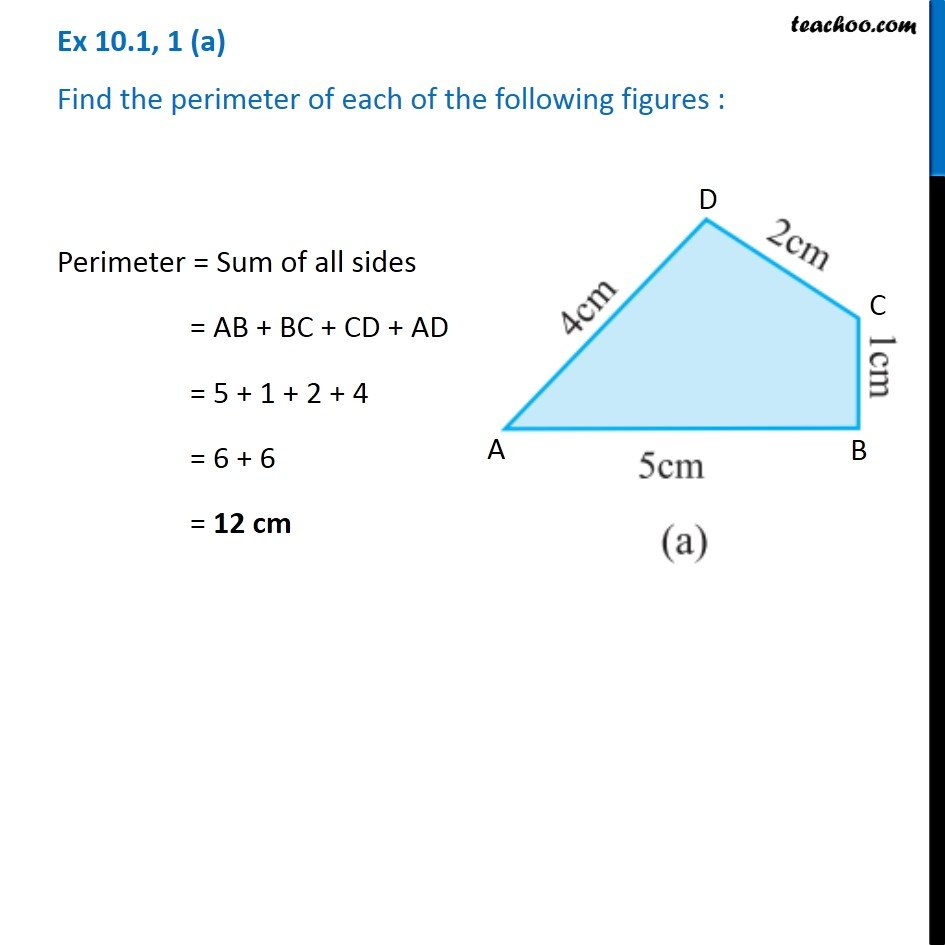Perimeter

Chapter 10 Class 6 Mensuration
Concept wiseIntroducing your new favourite teacher - Teachoo Black, at only ₹83 per month

### Transcript

Ex 10.1, 1 (a) Find the perimeter of each of the following figures : Perimeter = Sum of all sides = AB + BC + CD + AD = 5 + 1 + 2 + 4 = 6 + 6 = 12 cm